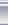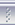# 力为的技术博客联系聚合管理#include <iostream>#define BOY_COUNT (5)bool gotByOneBoy(int& nTotal){--nTotal; // throw oneif(nTotal%5 != 0)return false;nTotal
-= nTotal/5// hide hisreturn true;}int _tmain(int argc, _TCHAR* argv[]){int n = 100;for(; n<10000++n){bool bFind = true;int nCur = n;std::cout
<< nCur << std::endl;for(int nBoy=1; nBoy<=BOY_COUNT; ++nBoy){bFind
= bFind && gotByOneBoy(nCur);}if(bFind){std::cout
<<  "This is the result: " << n << std::endl;break;}}char ch;std::cin
>> ch;return 0;}
posted on 2007-06-25 18:07 力为 阅读(2364) 评论(15)  编辑 收藏 引用 所属分类: Tools### 评论

# re: 五个小矮人分桃子-一道小学生的作业题目 2007-06-25 20:35 小不点

<---

猴子把多的一个扔入海中，拿走了一份。第二只猴子把剩下的桃子又平均分成五份，又多了
一个，它同样把多的一个扔入海中，拿走了一份，第三、第四、第五只猴子都是这样做的，
问海滩上原来最少有多少个桃子？
--->

回复  更多评论

# re: 五个小矮人分桃子-一道小学生的作业题目 2007-06-25 21:08 xlander

回复  更多评论

# re: 五个小矮人分桃子-一道小学生的作业题目 2007-06-25 21:09 小不点

#include <iostream>
using namespace std;

int main()
{
int total = 0;
int i = 0;

for(total = 1 ; total < 10000; total++)
{
for(; i < 5; i++)
{
if((total - 1) % 5 == 0)
{
total = (total-1)/5;
}
else
{
break;
}
}

if(i == 5)
{
for(i = 0; i < 5; i++)
{
total = total * 5+1;
}
cout << "The least total is : " << total << endl;
break;
}
}

return 0;
}  回复  更多评论

# re: 五个小矮人分桃子-一道小学生的作业题目 2007-06-25 22:57 力为

# re: 五个小矮人分桃子-一道小学生的作业题目 2007-06-26 09:26 Rocky

# re: 五个小矮人分桃子-一道小学生的作业题目 2007-06-26 12:16 闲耘
see http://blog.xianyun.org/2007/06/cpp-5.html

int getTotal(){
int total = 1; // 设初始野果总数1个
while (true){
int _t = total;
for (int i = 1; i <= 5; i++){
if ((_t - 1) % 5 == 0){ // 条件：扔掉1个能够5等分
_t = (_t - 1) / 5 * 4; // 第i个矮人拿走1/5后剩下的继续循环
if (i == 5){ // 如果5个矮人都拿到野果
}
}
}
total ++; // 不满足则野果总数加1
}
}

# re: 五个小矮人分桃子-一道小学生的作业题目 2007-07-01 17:45 s
5+1
30+1
155+1
780+1
3905+1
3906

回复  更多评论

# re: 五个小矮人分桃子-一道小学生的作业题目 2007-08-21 17:39 van
30个藏起来一份就是24,扔掉一个怎么会可以分成5份呢..  回复  更多评论

# re: 五个小矮人分桃子-一道小学生的作业题目 2007-09-03 16:56 haha

//4 3 2 1 0
#define M 5
#define N 1
int main(void)
{

int i ;
int sum=N;
for(i=0;i<M ; i++)
{
sum = sum*5 +1;
}
cout<<sum<<endl;

return 0;
}
3906个？！？  回复  更多评论

# re: 五个小矮人分桃子-一道小学生的作业题目 2007-10-08 00:22 biogerm
T = 5（5（5（5（5n+1)+1)+1)+1)+1

n = 1

T = 3906  回复  更多评论

# re: 五个小矮人分桃子-一道小学生的作业题目 2007-10-08 10:06 Icat

1.
#include <iostream>
using namespace std;
int main()
{
unsigned long x=0;//总数为X*5+1
while((((x*5+1)*256-2101))%(5*625))
{
x+=1;
}
cout<<x*5+1<<endl;
}
2.
#include <iostream>
using namespace std;
int main()
{
unsigned long lTotal=1;
unsigned long x=1;
int iMonkey=5;
//cin>>iMonkey;
bool bOK=false;
do
{
lTotal=x*iMonkey+1;
for(int i=1;i<iMonkey;++i)
{
lTotal=(lTotal+1)*(iMonkey)/(iMonkey-1);
if((lTotal-1)%(iMonkey))
break;
else
if(i==(iMonkey-1))bOK=true;
}
++x;

}while(!bOK);
cout<<y<<endl;
}

3121

回复  更多评论

# re: 五个小矮人分桃子-一道小学生的作业题目 2007-10-08 21:04 Bill Gates

# re: 五个小矮人分桃子-一道小学生的作业题目 2010-04-08 21:55 看看哪个方法好一点
#include <iostream>

bool test(int total, int level)
{
if (level==0)
return true;

if ((total-1)%5!=0)
return false;

total=(total-1)/5 *4;
return test(total,level-1);
}

bool test2(int iGet,int level,int& beforGet)
{
beforGet=iGet*5+1;
if (level==5)
return true;

if ((beforGet%4)!=0)
return false;

iGet=beforGet/4;
return test2(iGet,level+1,beforGet);
}

int main(int argc,char*argv[])
{
int i=1;
int total;
for (;i<10000 ;++i)
{
if (test2(i,1,total))
{
std::cout<<total<<std::endl;
// break;
}
}
for (i=0;i<10000 ;++i)
{
if (test(i,5))
{
std::cout<<i<<std::endl;
// break;
}
}

return 0;
}
回复  更多评论

# re: 五个小矮人分桃子-一道小学生的作业题目 2010-10-17 21:04 666

回复  更多评论

# re: 五个小矮人分桃子-一道小学生的作业题目 2010-12-06 16:49 wu4long

a1 = (a-1)/5
a2 = (4a1 -1)/5
a3 = (4a2 -1)/5
a4 = (4a3-1)/5
a5 = (4a4-1)/5

a = a1 + a2 + a3 + a4 + a5 + 5 + 4a5

a = 12 a5 + 8 + 53(a5 + 1)/256

a5 = 256* 1 -1 = 255
a = 12 * a5 + 8 + 53(a5+1)/256 = 3121.

回复  更多评论